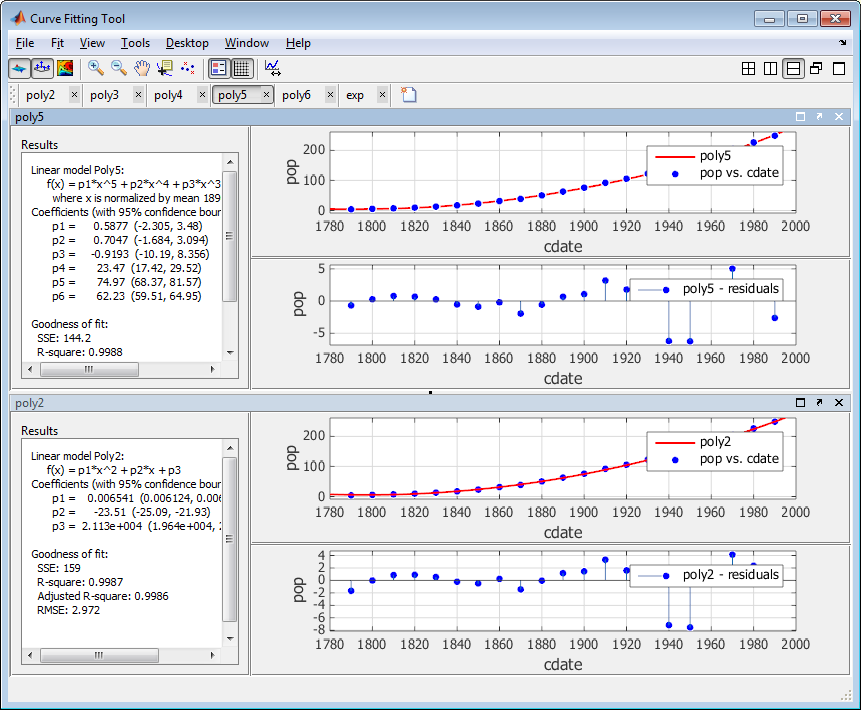# Video tutorial on Curve Fitting in MATLAB

## DescriptionThe curve fitting toolbox in MATLAB software is a ready-made tool for fitting curves and data surfaces. In this toolbox, you can analyze data, preprocess and post-process data, compare candidate models, and remove outlier.

In this toolbox, you can perform regression analysis using ready-made linear and nonlinear models, or create your own model.

In this toolbox, you can use optimized solvers to improve the quality of the fit. This toolkit also includes non-parametric modeling techniques such as interpolation and smoothing and splines.

After building the model, you can apply various post-processing methods to your model.In short, the curve fitting toolbox in MATLAB has the following features:

• Curve fitting for curves and surfaces
• Linear and nonlinear regression with custom equations
• A library of regression models with optimized starting points and solver parameters
• Interpolation methods include B-spline, plane spline, and tensor-product spline
• Smoothing techniques include smoothing spline and centralized regression and Savitzky-Golay filters and moving averages
• Pre-processing methods include outlier removal, segmentation, resizing, and weighting of data
• Post-processing methods include internalization and extrapolation, and integral and derivative confidence intervals.

## Reviews

There are no reviews yet.